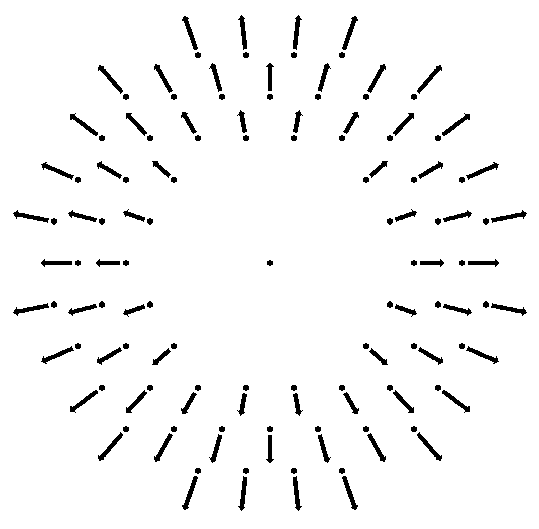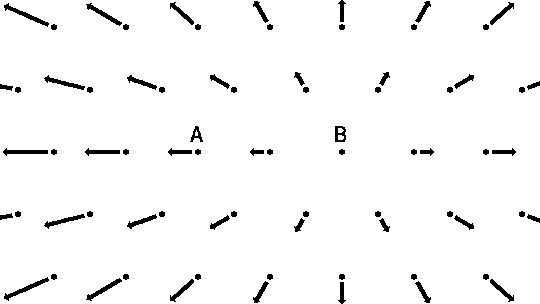# Where was the center of the Big Bang?

The Big Bang has no center. It is not an explosion radiating from a point. In an explosion you get an expanding spherical shell of fragments as shown below:This shell has both an outer and an inner edge, and these can be used to locate the position of the explosion. But in the Big Bang there is no edge. The Figure below shows a galaxy A and the velocities measured by observers on A:There is no edge in this figure except for the arbitrary cropping that I have done to make it fit on the page. You might think that by backtracking the velocity vectors you can locate the center of the Big Bang (and it is at A) but this is not the case! Consider the velocities measured by observers on galaxy B: since they can only measure relative velocities, the velocities seen by B are the vector difference between the velocity seen by A and the velocity of B as seen by A. The figure below shows these velocities relative to B which are measured by observers on galaxy B:The observers at B see exactly the same pattern of velocities the observers at A do. You can prove this by printing out two copies of the page and if you flip one of them over can can line up A's view and B's view precisely. But you can't have both A and B being the center! There is no center because all positions in the Universe are equivalent. The Universe is homogeneous, which is part of the cosmological principle.